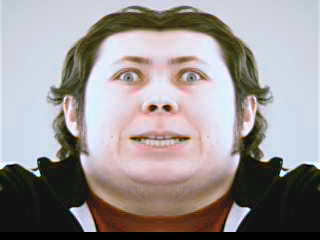# Playing with OpenCV

## February 26, 2008

OpenCV is a cross platform video library I’ve been playing with. Today I coded up a horizontal mirror effect, took about 30 minutes. I worked out all the byte manipulations on a piece of paper, that took the longest. Coding was a breeze with OpenCV, and I tried out some of the built in effects too, stacking them on top of each other.

Here’s my first version source for the mirror effect, it’s rough since I just translated what I had written down into code. “frame” is a captured IplImage.

```int halfsies = frame->width/2;
for(int i = 0; i < frame->height; i++) {
int offset = i*frame->width*3;
for(int j = 0; j < halfsies; j++) {
frame->imageData[offset+(frame->width*3-1)-2-(j*3)] = frame->imageData[offset+(j*3)];
frame->imageData[offset+(frame->width*3-1)-1-(j*3)] = frame->imageData[offset+(j*3)+1];
frame->imageData[offset+(frame->width*3-1)-(j*3)] = frame->imageData[offset+(j*3)+2];
}
}```

Here is the reformed version, cleaner by far.

```int halfFrame = frame->width/2;
int frameBytes = frame->width*3-1;
for(int i = 0; i < frame->height; i++) {
int offset = i*frame->width*3;
for(int j = 0; j < halfFrame; j++) {
int jBytes = offset+frameBytes-(j*3);
int ojBytes = offset+(j*3);
frame->imageData[jBytes-2] = frame->imageData[ojBytes];
frame->imageData[jBytes-1] = frame->imageData[ojBytes+1];
frame->imageData[jBytes] = frame->imageData[ojBytes+2];
}
}```

And here is what it looks like. The first one is without any other effects, the second is with the OpenCV effect “erode”.You can get the full source of my fxTest.cpp here if you want it.

The Introduction to programming with OpenCV was a great resource for me.

2.omid says: